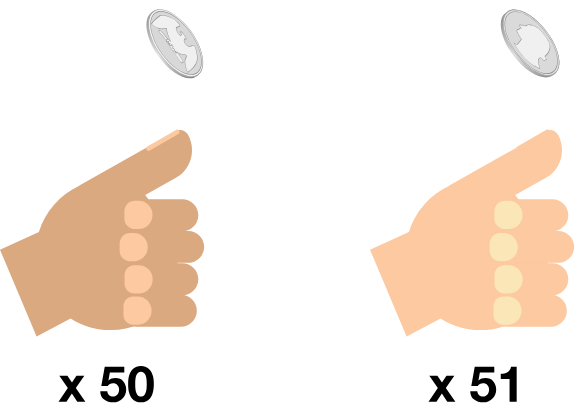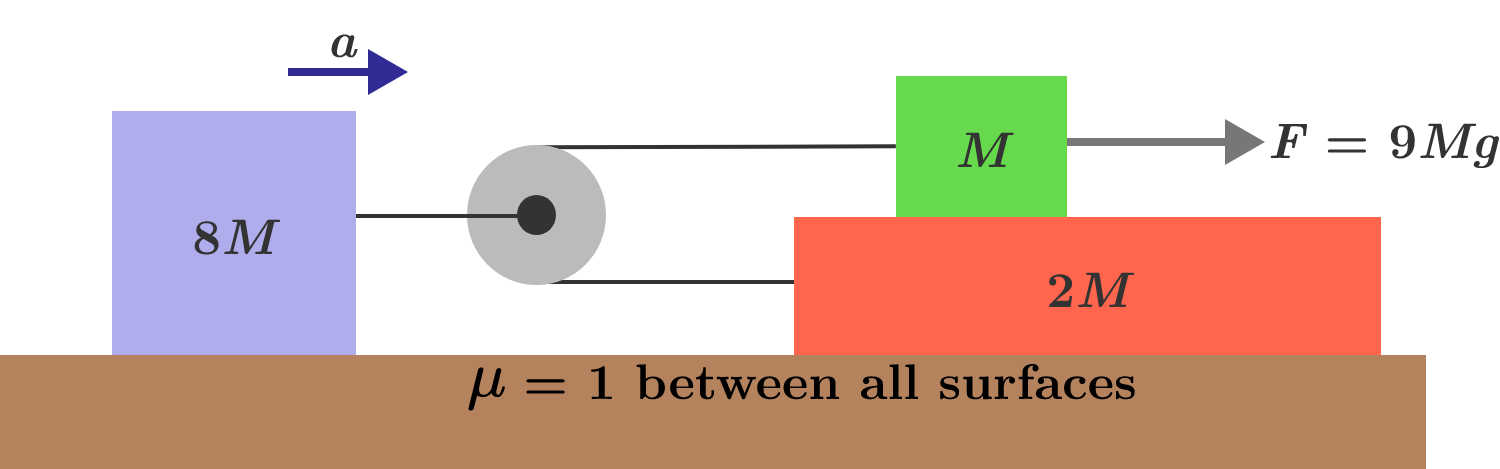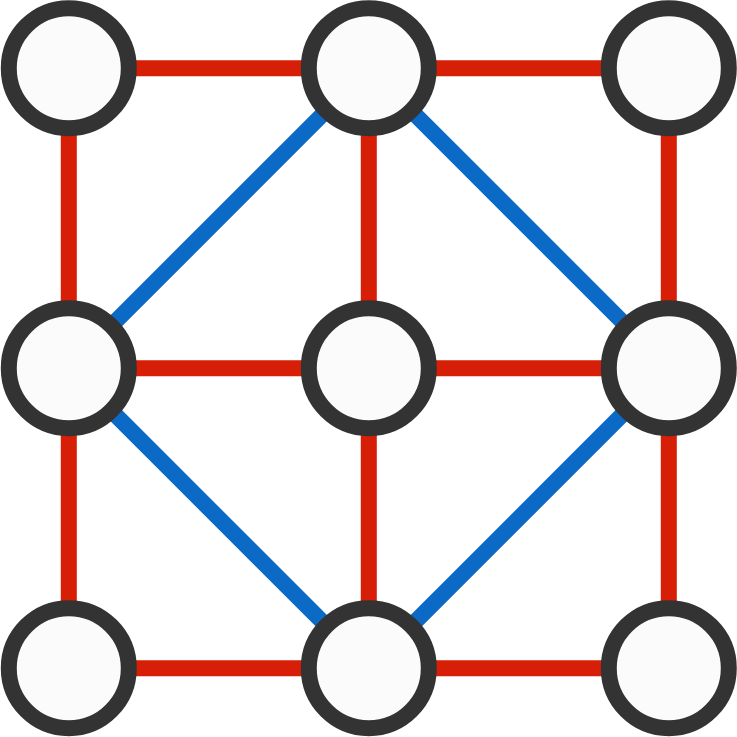# 2018-12-10 Intermediate

Ana tosses a fair coin 50 times. Bastian tosses another fair coin 51 times.

What's the probability that Bastian gets more heads than Ana does?I'm looking for a positive integer $x$ with $n$ positive factors such that the number of positive factors in $x^2$ is $n^2.$

One answer is 1.

Are there any other answers?

In the system below, the coefficient of friction between all surfaces is the same and equal to 1. When you apply force $F$ on the green body, the three bodies move with different accelerations with respect to the ground.

What is the acceleration of the blue body?$p,q,r,s$ are the 4 roots of the equation

$x^4 - ax^3 +ax^2 + bx +c =0.$

What is the minimum possible value of $p^2+q^2+r^2+s^2?$

Note:

• $p,q,r,s$ are not necessarily real.
• $a,b,c$ are real coefficients.

In the figure below, there are 6 squares: 4 small, 1 medium (in blue), and 1 large. There are 9 circles, which will be filled with all the integers 1 through 9 such that the sum of the vertices of each square is 20.

What is the product of the 4 numbers along the blue square?×

Problem Loading...

Note Loading...

Set Loading...